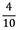## Mathematics 4

### Course Outline

#### Number

• read, write, represent, and describe whole numbers to 10 000 using concrete materials, pictures, expressions (e.g., 400 + 7), words, place-value charts, and symbols
• describe numbers to 10 000 in two or more parts (partition)
• explore place value (ones, tens, hundreds, thousands) to 10 000
• compare and order whole numbers to 10 000
• represent and describe decimal numbers (tenths and hundredths) using concrete materials, money, pictures, expressions, words, and symbols
• read, write, and recognize decimal number symbols (to hundredths)
• compare and order decimal numbers (tenths and hundredths)
• name and record fractions of a set and fractions of one whole
• learn to use common fractions and decimal numbers to describe real-life situations
• represent and describe fractions using concrete materials, pictures, and symbols
• compare fractions using concrete materials, pictures, words, and symbols
• understand and show the relationship between decimals and fractions (an also be represented as 0.4)
• explain why different representations of the same number are equal
• estimate sums, differences, products, and quotients
• explain the reasons for our estimates
• choose appropriate estimates
• solve addition and subtraction questions mentally using a variety of strategies
• add and subtract whole numbers
• solve and create story problems that add and subtract whole numbers
• add and subtract decimal numbers (to hundredths)
• solve and create story problems that add and subtract decimal numbers (to hundredths)
• represent multiplication and division problems concretely, pictorially, and symbolically
• recall basic multiplication facts and determine basic division facts
• multiply two- or three-digit whole numbers by one-digit numbers using concrete models, pictures, symbols, and personal strategies
• divide one- and two-digit whole numbers by one-digit divisors using concrete models, pictures, symbols, and personal strategies
• ▪ solve and create story problems that multiply and divide whole numbers
• ▪ use a calculator for problems that involve larger whole numbers

#### Patterns and Relations

• identify and describe (orally and in writing) patterns
• identify and describe patterns found in charts and tables, such as the multiplication table
• determine missing elements in a pattern, table, and chart
• identify errors in a pattern, table, and chart
• create a table or chart from a pattern shown concretely
• use information in a problem to create a table or chart
• create a pattern concretely from a table or chart
• explain why the same pattern exists whether it is shown concretely, in a table, or in a chart
• use patterns in tables or charts to solve problems
• use diagrams (Carroll diagrams and Venn diagrams) to sort and organize information and to solve problems
• describe the patterns and relationships shown in a given Carroll diagram or Venn diagram
• use a symbol to represent the unknown number in an addition, subtraction, multiplication, or division problem (e.g., 24 ÷ 6 =? 24 = 6 × ? or 24 = 6 × ◊)
• write an equation with an unknown to represent a given story problem
• create a story problem for a given equation having one unknown
• solve one-step equations having one unknown
• represent addition, subtraction, multiplication, and division situations with an equation having one unknown

#### Measurement

• identify the number of hours in a day
• read and write time from a 12-hour analog clock, a 24-hour analog clock, and a 24-hour digital clock
• describe time as “minutes to” or “minutes after” the hour
• describe time as a.m. or p.m. and identify activities that occur during the a.m. and/or during the p.m.
• read and write dates in a variety of forms (e.g., March 21, 2015; 2015/03/21; 21/03/2015; or 21/03/15)
• describe area as the measure of the space inside a 2-D shape
• identify personal referents for a square centimetre and a square metre
• identify standard units (square centimetre and square metre) for area
• estimate area in square centimetres and square metres
• determine the area of regular and irregular 2-D shapes in square centimetres and square metres
• recognize and demonstrate that different rectangles can have the same inside space (area)
• recognize and demonstrate that for any given area, many different rectangles are possible
• draw rectangles for a given area
• solve problems involving area

#### Geometry

• recognize, name, and describe rectangular prisms and triangular prisms
• sort prisms using the shape of the base
• construct rectangular and triangular prisms from their nets
• construct models for rectangular and triangular prisms using concrete materials
• identify rectangular and triangular prisms in the real world
• investigate rectangular and triangular prisms to see the differences in the number of vertices, edges, and faces of each
• determine if two 2-D shapes are congruent
• create congruent 2-D shapes
• identify congruent 2-D shapes shown in different positions
• identify lines of symmetry in 2-D shapes
• create symmetrical shapes
• find examples of symmetry in the real world
• determine if a 2-D shape is symmetrical
• complete a symmetrical drawing when given one-half of the shape

#### Statistics and Probability

• read and interpret bar graphs and pictographs
• construct bar graphs and pictographs
• explore real-world issues and collect data to answer questions that students have
• use one-to-one and many-to-one correspondence to display data